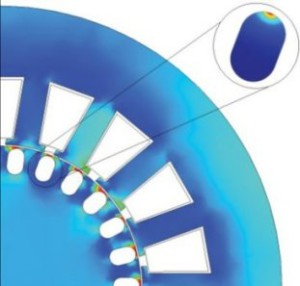Technology

Each device, each application, each idea, needs to be analyzed according to each particular requirement and unique environment. One solution doesn’t fit all.

As a scientist, you want to decide which field solver best suits your application. INTEGRATED’s field solvers have been proven in a diverse range of applications for over 25 years. Our Boundary Element Method (BEM) and Finite Element Method (FEM) solvers are available in the same package to allow you to select the best method for any problem. Now, you can independently verify the solution within one program, using two completely different solution techniques.

A significant side benefit of having both BEM and FEM solvers is the ability to check the validity of solutions using two completely different analysis methods.Current density contour plot in induction motor rotor bar

Finite elements solve by breaking up a problem into small regions. Solutions are found for each region taking into account only the regions that are right next to the one being solved. In the case of magnetic fields where FEM is often used, the vector potential is normally what is solved for in these regions.

Magnetic field solutions are derived from the vector potential through differentiating the solution. This can cause problems in smoothness of field solutions. Technically, any partial differential equation can be solved using FEM (although some types will do better than others).

The Finite Element Method is a numerical technique for solving models in differential form. For a given design, the FEM requires the entire geometry, including the surrounding region, to be modeled with finite elements. A system of linear equations is generated to calculate the potential (scalar or vector) at the nodes of each element. Therefore, the basic difference between these two techniques is the fact that BEM only needs to solve for unknowns on the boundaries, whereas FEM solves for unknowns in the volume.

While BEM can solve nonlinear problems, the nonlinear contribution requires a volume mesh. A volume mesh diminishes the benefits of BEM listed above. In fact, for a saturating nonlinear magnetic problem, the saturation characteristic is best solved with FEM.